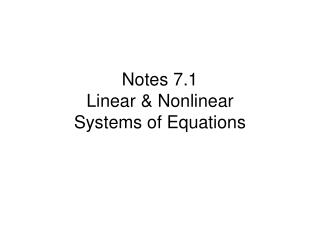DownloadDownload PresentationNotes 7.1 Linear & Nonlinear Systems of Equations

# Notes 7.1 Linear & Nonlinear Systems of Equations

Download Presentation## Notes 7.1 Linear & Nonlinear Systems of Equations

- - - - - - - - - - - - - - - - - - - - - - - - - - - E N D - - - - - - - - - - - - - - - - - - - - - - - - - - -
##### Presentation Transcript

1. Notes 7.1Linear & NonlinearSystems of Equations

2. The solution to a system of equations is the set of points which satisfy ALL the equations in the system. What does a solution look like graphically? Example: p. 503 #7:

3. Suppose we wish to solve a system algebraically. A good method is SUBSTITUTION: 1. Solve one of the equations for one variable in terms of the other (x = or y = ) 2. Substitute the expression found in step 1 into the other equation. Hooray! We now have an equation with only one variable! 3. Solve your nice one-variable equation you write in step 2 4. Back-substitute your answer from step 3 into the equation you first wrote in step 1 5. Check your answer by substituting these values into BOTH the original equations!!

4. Does EVERY system have a real solution?

5. Homework: p. 503 - 506 # 3, 7, 11, 13, 21, 39 43 - 47 (ODD), 61, 63, 69